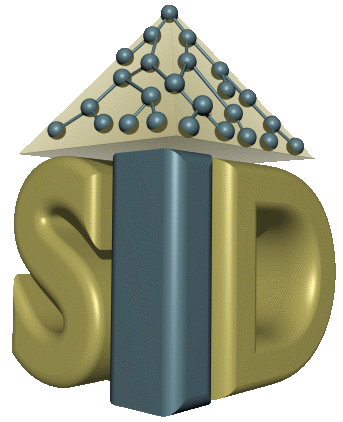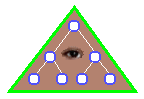﻿ Mapping Information GrammarOBSERVER

Mapping Information Grammar

```      <concept-mapping> ::= <basic-concept-mapping >
| <basic-concept-mapping> <concept-mapping>

<basic-concept-mapping> ::= <relation> [<list-of-attr>] [<list-of-types>]

<role-mapping> ::= <basic-role-mapping >
| <basic-role-mapping> <role-mapping>

<basic-role-mapping> ::= <relation> [<list-of-attr>] [<list-of-types>]
[<list-of-attr>] [<list-of-types>]  trans-function

<relation> ::= entity-name
| [ projection <relation> [<list-ofattr>] ]
| [ selection <relation> <condition> ]
| [ aggr-function <relation> <list-of-attr> [<aggr-function> <list-of-attr> ] ]
| [ join <relation> <relation> <join-condition> ]
| [ union <relation> <relation> ]
| [ intersection <relation> <relation> ]
| [ difference <relation> <relation> ]
| [ division <relation> <relation> ]

<list-of-attr> ::= [ attr-name
| attr-name [<list-of-attr>] ]

<condition> ::= [ not <condition> ]
| [ and <condition> <condition> ]
| [ or <condition> <condition> ]
| [ is-null attr_name ]
| [ > <term> <term> ]
| [ < <term> <term> ]
| [ => <term> <term> ]
| [ <= <term> <term> ]
| [ = <term> <term> ]
| [ <> <term> <term> ]

<term> ::= attr_name
| constant

<join-condition> ::= [ and <join-condition> <join-condition> ]
| [ < attr_name attr_name ]
| [ > attr_name attr_name ]
| [ => attr_name attr_name ]
| [ <= attr_name attr_name ]
| [ = attr_name attr_name ]
| [ <> attr_name attr_name ]

<aggr_function> ::= count
| avg
| sum
| max
| min

<list-of-types> ::= [ type-name
| type-name [<list-of-types>] ]
```